# JP3966866B2 - Imaging apparatus, camera, and signal processing method - Google Patents

## Info

Publication number
JP3966866B2
JP3966866B2 JP2004117670A JP2004117670A JP3966866B2 JP 3966866 B2 JP3966866 B2 JP 3966866B2 JP 2004117670 A JP2004117670 A JP 2004117670A JP 2004117670 A JP2004117670 A JP 2004117670A JP 3966866 B2 JP3966866 B2 JP 3966866B2
Authority
JP
Japan
Prior art keywords
color signal
green
red
value
means
Prior art date
Legal status (The legal status is an assumption and is not a legal conclusion. Google has not performed a legal analysis and makes no representation as to the accuracy of the status listed.)
Active
Application number
JP2004117670A
Other languages
Japanese (ja)
Other versions
JP2005303704A (en
Inventor

Original Assignee

Priority date (The priority date is an assumption and is not a legal conclusion. Google has not performed a legal analysis and makes no representation as to the accuracy of the date listed.)
Filing date
Publication date
Application filed by 三菱電機株式会社 filed Critical 三菱電機株式会社
Priority to JP2004117670A priority Critical patent/JP3966866B2/en
Publication of JP2005303704A publication Critical patent/JP2005303704A/en
Application granted granted Critical
Publication of JP3966866B2 publication Critical patent/JP3966866B2/en
Application status is Active legal-status Critical
Anticipated expiration legal-status Critical

## Images

•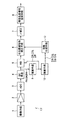•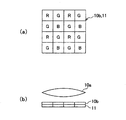•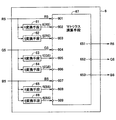•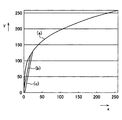•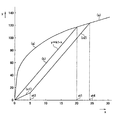•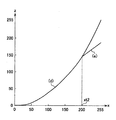•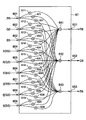•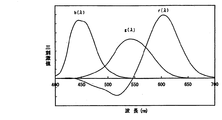•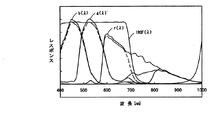•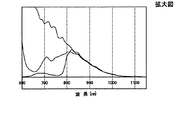•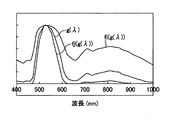•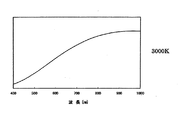•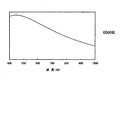•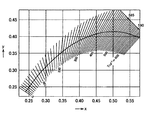•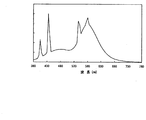•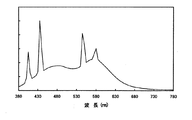•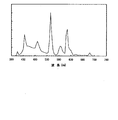•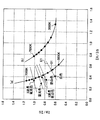•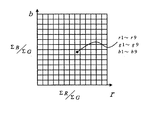•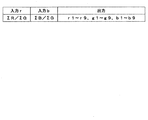•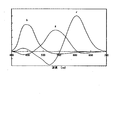•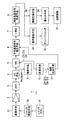## Description

The present invention relates to an image pickup apparatus, and more particularly to an image pickup apparatus including a signal processing unit that performs relative visibility correction without using an infrared removal filter (IRCF). The present invention also relates to a camera provided with such an imaging apparatus. The present invention further relates to a signal processing method in such an imaging apparatus.

A conventional imaging device has a lens that forms incident light, an imaging device that converts an optical image formed by the lens into an electrical signal, and a signal process performed on the electrical signal obtained from the imaging device to obtain a predetermined signal. Signal processing means for obtaining an image signal.
When an image pickup apparatus is configured with only one CCD (Charge Coupled Device) sensor or CMOS (Complementary Metal Oxide) sensor used as a normal image sensor, that is, in a single-plate sensor, a pixel filter is used as a color filter for color separation. Different colors are provided on the sensors.
In order to obtain red (R), green (G), and blue (B) color signals, R, G, and B primary color filters that transmit light bands corresponding to R, G, and B are used. In some cases, magenta (Mg), cyan (Cy), yellow (Ye), and G complementary color filters are used. Each of the above color filters is designed to have a spectral transmission characteristic so as to transmit a target color using a dye or pigment, but has a certain transmittance even in the near infrared region. Further, since the photoelectric conversion unit of the image sensor is mainly composed of a semiconductor such as silicon (Si), the spectral sensitivity characteristic of the photoelectric conversion unit is sensitive to near infrared light having a long wavelength. Therefore, the signal obtained from the image sensor provided with the color filter also reacts to light in the near infrared region.

On the other hand, the color vision characteristic, which is a sensitivity characteristic for human colors, and the relative visual sensitivity characteristic, which is a sensitivity characteristic for brightness, are sensitivity characteristics from 380 nm to 780 nm, which are referred to as the visible range, and are almost in the wavelength range longer than 700 nm. Does not have. Therefore, in order to match the color reproducibility of the image pickup device with human color vision characteristics, an infrared ray removal filter for correcting visibility (hereinafter referred to as IRCF: Infrared Cut Filter) that does not allow light in the near infrared region to pass in front of the image pickup device. It was necessary to provide.

On the other hand, in the case where sensitivity is more important than color reproducibility, for example, in a surveillance camera, it is better to make the imaging device receive near infrared light without providing IRCF in order to use light in the near infrared region.

Therefore, when color reproducibility is required, an IRCF is installed in front of the image sensor, and when priority is given to sensitivity, a mechanism means for moving the IRCF is provided to receive near-infrared rays. Various techniques have been proposed in which an IRCF is provided in a part of a diaphragm for adjusting the amount of IR, and the IRCF is installed or removed in front of an image sensor in accordance with the amount of light (see, for example, Patent Document 1).
Further, white balance is taken without installing the IRCF, and sensitivity is improved by generating a luminance signal with a color mixture ratio different from the color mixture ratio of the R, G, and B signals that generate the luminance signal when the IRCF is installed. Technology has also been proposed (see Patent Documents 2 and 3).

JP 2001-36807 A JP 2003-134522 A JP 2003-264843 A

However, the conventional imaging device described in Patent Document 1 requires a mechanism means for moving the IRCF, which is disadvantageous for downsizing of the unit including the imaging element, and is simple in performing light amount adjustment using the electronic shutter of the imaging element. Since typical imaging devices (for example, PC cameras (PC cameras), mobile phone cameras, toy cameras (TOY cameras), consumer surveillance cameras) often do not have an aperture mechanism, a new IRCF is attached and detached. Mechanism means must be provided.

The imaging devices described in Patent Documents 2 and 3 have no problem when obtaining a black and white video signal, but when obtaining a color video signal, the color signal only adjusts the white balance, and the luminance signal is also human. Since the color signal ratio does not take into account the relative visual sensitivity characteristic of the color signal, the video signal of the color has the R, G, B value obtained by the human color vision characteristic or the spectral sensitivity characteristic obtained by linearly converting it. Different video signals, that is, color video signals are video signals having a large color difference ΔE * ab (JIS Z8730) with respect to R, G, and B values obtained by human color vision characteristics or spectral sensitivity characteristics obtained by linearly converting the color vision characteristics. Thus, accurate color reproducibility cannot be obtained.
In addition, there is a problem that an image with a good S / N (signal to noise ratio) cannot be obtained, and that good color reproducibility cannot be obtained under various light sources including artificial light such as a fluorescent lamp. .

The present invention has been made in order to solve the above-described problems, and obtains a color video signal with good color reproducibility even when near infrared light is incident without using IRCF. An object of the present invention is to provide an image pickup apparatus and a signal processing method capable of obtaining an image with good S / N and obtaining good color reproducibility under various light sources including artificial light such as a fluorescent lamp. .

The present invention
Color signal generating means for receiving incident light and outputting first red, green, and blue color signals corresponding to the incident light;
Spectral sensitivity characteristic correcting means for generating a second red, green, blue color signal by performing a matrix operation including multiplication of the first red, green, blue color signal and a coefficient;
The spectral sensitivity characteristic correcting means includes:
The first red, green, and blue color signals and the first red, green, and blue color signals have values approximated to values obtained by raising a predetermined first constant as an exponent. Red having a value approximated to a value obtained by exponentiating the third color signal of red, green, and blue and the first red, green, and blue color signal with a predetermined second constant as an exponent A matrix operation including the fourth color signal of green, blue, the multiplication of the coefficients for these signals, and the mutual addition of the results of the multiplication,
The first constant, the second constant, and the coefficient are obtained by a human color vision characteristic or a linear conversion of the color characteristic generation means and the spectral sensitivity characteristic correction means. It is approximated to the spectral sensitivity characteristic, and is defined to correct the response characteristic in the near infrared region of the color signal generating means,
Before Symbol color signal generating means, red obtained from the imaging unit, green, is integrated over a plurality of pixels in a screen No. Iroshin blue, integration of the color signal integrated value ΣR and blue red color signal Integrating means for obtaining the value ΣB and the integrated value ΣG of the green color signal ;
The integrated value ΣR of the red color signal, the integrated value ΣB of the blue color signal, and the integrated value ΣG of the green color signal obtained by the integrating means are obtained, and these ratios ΣR / ΣG and ΣB / ΣG are obtained. A division means to calculate;
The image pickup apparatus further includes: coefficient generation means for generating the coefficient used by the spectral sensitivity characteristic correction means in accordance with the values of the ratios ΣR / ΣG and ΣB / ΣG.

According to the present invention, good color reproducibility can be obtained without using IRCF, and an image with good S / N can be obtained. Furthermore, good color reproducibility can be obtained under various light sources including artificial light such as a fluorescent lamp, and an imaging device that does not require an IRCF can be obtained.

Embodiment 1 FIG.
FIG. 1 is a schematic configuration diagram showing an imaging apparatus according to Embodiment 1 of the present invention. As shown in the figure, this imaging apparatus includes an imaging means 1, an amplifying means 2, an A / D converter (ADC) 3, a direct current component reproducing means (DC reproducing means) 4, a white balance means 5, and a spectroscopic device. It includes a sensitivity characteristic correction unit 6, a gamma (γ) correction unit 7, and a luminance / color difference signal generation unit 8. Furthermore, it has a dividing means 12 and a coefficient generating means 13.

For example, as shown in FIGS. 2A and 2B, the image pickup means 1 includes a lens 10a, an image pickup element 11 having a plurality of photoelectric conversion elements arranged two-dimensionally, and a color filter as a color separation means. 10b.
The plurality of photoelectric conversion elements of the image sensor 11 correspond to the three primary colors of red (R), green (G), and blue (B) arranged in a Bayer type as shown in FIG. 2A, for example. Is covered with a color filter 10b having a spectral transmittance.

The light incident from the lens 10 a forms an image on the light receiving surface of the image sensor 11. As described above, the image sensor 11 is covered with the color filter 10b, and from each photoelectric conversion element, color components corresponding to the spectral transmittance of the color filter 10b, that is, R, G, B analog video signals R1, G1 and B1 are output.

In this way, the R, G, B analog signals output from the image pickup means 1 (hereinafter may be referred to as “R signal”, “G signal”, and “B signal”, respectively) are amplified by the amplification means 2. Amplified. The video signal output from the amplifying means 2 is converted into a digital signal by the ADC 3. The DC level of the video signal converted into the digital signal is reproduced by the DC reproducing means 4. In DC reproduction, the offset level held before A / D conversion by the ADC 3 is DC-shifted or clamped so that the black level of the normal video signal becomes “0”.

The integrating unit 9 integrates the R signal, G signal, and B signal output from the DC reproducing unit 4 over a plurality of pixels, for example, over the entire screen, and the integrated values ΣR, G of the R signal are integrated. An integrated value ΣG and an integrated value ΣB of the B signal are obtained.

Next, the white balance (WB) means 5 has three amplifying means (not shown) for amplifying the R, G, B signals, respectively, and receives the R, G, B signals from the DC reproducing means 4, Further, the integrated values ΣR, ΣG, and ΣB are received from the integrating means 9, and the R, G, and B signal values of the white portion of the subject become equal according to the imaging condition of the video signal (that is, the white portion is achromatic) Is applied). Normally, the white balance means 5 is based on Evans' principle that the accumulated color for all pixels in the screen of a general subject is an achromatic color, so that the integrated values ΣR, ΣB, and ΣG are equal to each other. White balance is performed by changing the gain of the amplification means.

The imaging means 1, amplification means 2, ADC 3, DC reproducing means 4, white balance means 5, and integrating means 9 receive incident light and output R, G, B color signals corresponding to the incident light. Color signal generation means 20 is configured.

The dividing means 12 receives the integrated value ΣR of the R signal, the integrated value ΣB of the B signal, and the integrated value ΣG of the G signal from the integrating means 9, and the ratio ΣR / ΣG and ΣB between these integrated values. / ΣG is calculated and output.

The coefficient generation unit 13 determines a coefficient as described later based on the output of the division unit 12.
The coefficient generating means 13 may calculate and output a coefficient according to the ratio of the integrated values obtained by the dividing means 12, or may store several sets of constants in advance, Accordingly, an optimum one may be selected and output from them.
As will be described later, the matrix calculation unit 67 of the spectral sensitivity characteristic correction unit 6 performs matrix calculation using the coefficients output from the coefficient generation unit 13.

The spectral sensitivity characteristic correction unit 6 receives the white balance R, G, B signals R5, G5, and B5 output from the white balance unit 5, and performs matrix calculation (to be described later) of the respective signals to perform the matrix calculation described later. Color signals R6, G6, and B6 are output in which the influence on the color reproducibility due to the sensitivity characteristics in the near infrared region is corrected.

The gamma correction unit 7 performs non-linear gradation conversion on the video signals R6, G6, and B6 output from the spectral sensitivity characteristic correction unit 6.

The luminance color difference signal generation means 8 converts the R, G, B signals R7, G7, B7 output from the gamma correction means 7 into a luminance signal (Y signal) and two color difference signals (Cr signal, Cb signal). In this conversion (YCrCb conversion), the luminance / chrominance signal generation means 8 performs a linear matrix operation of the following equation (2), which is usually multiplied by a coefficient matrix of 3 rows and 3 columns, to generate Y, Cr, and Cb signals. .

In the formula (2), the matrix coefficient of 3 rows and 3 columns is defined by, for example, IEC (International Electrotechnical Commission) 61966-2-1), y1 = 0.2990, y2 = 0.5870, y3 = 0 1140, cr1 = −0.1687, cr2 = −0.3313, cr3 = 0.5000, cb1 = 0.5000, cb2 = −0.4187, cb3 = −0.0813.

The configuration and principle of the spectral sensitivity characteristic correcting means 6 will be described below.
The spectral sensitivity characteristic correction means 6 is such that the overall characteristics of the color signal generation means 20 and the spectral sensitivity characteristic correction means 6 approximate human color vision characteristics or spectral sensitivity characteristics obtained by linearly transforming them. This is for correcting the response characteristic in the near infrared region of the color signal generating means.

FIG. 3 is a schematic configuration diagram showing the configuration of the spectral sensitivity characteristic correcting means 6. The R, G, B color signals R5, G5, B5 output from the white balance means 5 are input to the spectral sensitivity characteristic correction means 6. The spectral sensitivity characteristic correcting unit 6 performs multiplication of the fi conversion units 61, 63, and 65, the fj conversion units 62, 64, and 66 with each input signal and a coefficient for each, and adds the multiplication results to three rows. 9-column matrix calculation means 67.

The fi conversion means 61, 63, and 65 output values approximate to values obtained by exponentiation with a predetermined real number i smaller than 1 for the input signals R5, G5, and B5. And the fi conversion means 61, 63, 65 generally (ie, in most areas of the range that the input can take), more specifically, close to the minimum value of the range that the input can take. In a region other than the region, it has a value obtained by exponentiating the real number i with respect to the input signals R5, G5, and B5, and when the input signal is close to zero, it is obtained by the above power. A signal having a value limited to a value smaller than the value is output. For example, in the range where the input signal is close to zero, the ratio of the output signal to the input signal is limited to a predetermined value or less.
The fj conversion means 62, 64, and 66 output values approximate to values obtained by exponentiation with a predetermined real number j greater than 1 for the input signals R5, G5, and B5. And the fj conversion means 62, 64, 66 are generally (ie, in most areas of the range that the input can take), more specifically, close to the maximum value of the range that the input can take. In a region other than the region, the input signal R5, G5, B5 has a value obtained by exponentiation of the real number j as an exponent, and in the range where the input signal is close to the maximum value, it is obtained by the above power. A signal having a value limited to a value smaller than a given value is output. For example, in the range where the input signal is close to the maximum value, the ratio of the output signal to the input signal is limited to a predetermined value or less.

The input / output characteristics of the three fi conversion units 61, 63, and 65 are the same, and an example is shown in FIGS. In FIG. 4, the horizontal axis indicates the input x (x = R5, G5 or B5), and the vertical axis indicates the output y (y = fi (R5), fi (G5) or fi (B5)). FIG. 5 is an enlarged view of a small input / output portion of FIG.
For example, when the first real number i is 0.3, the fi conversion means 61, 63, 65 are generally more specific (that is, in most of the possible range of input). Outputs a value proportional to the 0.3th power of the input signal x in a region other than the region close to the minimum value in the possible range of the input, but the input signal x is a predetermined value close to 0 In a range smaller than xt1, the value is limited to a value smaller than 0.3, for example, k1 · x. Here, k1 is a constant, and is set to “6”, for example. Xt1 is “20”, for example.
4 and 5, x to the power of 0.3 is indicated by a curve (a). In these figures, the minimum value that the input signal x can take is “0 (zero)”, the maximum value is “255”, and the minimum value that the output signal y can take is “0 (zero)”. The maximum value is “255” assuming 8 bits, and the relationship of output to input is the relationship between values normalized by the maximum value. That is, between x and y in the figure,
(Y / 255) = (x / 255) 0.3
There is a relationship.
Further, k1 · x is indicated by a straight line (b).
At x = xt1, the straight line (b) and the curve (a) intersect.
As a result, in the range smaller than x = xt1, the output y is k1 · x, and in the range where x is greater than or equal to xt1, the value obtained by normalizing the output y is the 0.3th power of the value obtained by normalizing x. It becomes.

The input / output characteristics of the three fj conversion means 62, 64, and 66 are the same, and an example is shown in FIG. In FIG. 6, the horizontal axis indicates the input x (x = R5, G5 or B5), and the vertical axis indicates the output z (z = fj (R5), fj (G5) or fj (B5)).
For example, when the above second real number j is 2, the fj conversion means 62, 64, 66 generally (ie, in most of the range that the input can take), more specifically, A value proportional to the square of the input signal x is output in a region other than the region close to the maximum value in the range that the input can take, but the input signal x is larger than a predetermined value xt2 close to the maximum value. In a large range, the value is limited to a value smaller than the square of x, for example, k2 · x. Here, k2 is a constant and is set to 1.5, for example. Xt2 is “200”, for example.
In FIG. 6, the square of x is indicated by curve (a). In this figure, the minimum value that the input signal x can take is “0 (zero)”, the maximum value is “255”, the minimum value that the output signal z can take is “0 (zero)”, and the maximum value is “255”, and the relationship of the output to the input is a relationship between values normalized by the maximum value. That is, between x and z in the figure,
(Z / 255) = (x / 255) 2
There is a relationship.
Further, k2 · x is indicated by a straight line (b).
At x = xt2, the straight line (b) and the curve (a) intersect.
As a result, in the range where x is greater than xt2, the output z is k2 · x, and in the range where x is equal to or less than xt2, the value obtained by normalizing the output z is the square of the value obtained by normalizing x.

In the following description, the conversion by the fi conversion means 61, 63, 65 may be simply referred to as “fi conversion”, and the conversion by the fj conversion means 62, 64, 66 may be simply referred to as “fj conversion”.

The R signal R5 is input as it is to the input terminal 601 of the matrix calculation means 67, and is also input to the fi conversion means 61 and the fj conversion means 62, and these outputs (fi (R5), fj (R5)) are matrix calculation means. It is input to 67 input terminals 602 and 603.
Similarly, the G signal G5 is directly input to the input terminal 604 of the matrix calculation means 67 and also input to the fi conversion means 63 and the fj conversion means 64, and these outputs (fi (G5), fj (G5)) are obtained. The data is input to input terminals 605 and 606 of the matrix calculation means 67.
Similarly, the B signal B5 is input as it is to the input terminal 607 of the matrix calculation means 67, and is also input to the fi conversion means 65 and the fj conversion means 66, and these outputs (fi (B5), fj (B5)). The data is input to input terminals 608 and 609 of the matrix calculation means 67.

The matrix calculation means 67 performs a matrix calculation by multiplying a matrix coefficient of 3 rows and 9 columns, performs a matrix calculation using a coefficient matrix of 3 rows and 9 columns, and performs R, G, and B color signals R6, G6, B6 is generated. The R, G, and B signals R6, G6, and B6 obtained by the calculation are output from terminals 651, 652, and 653, respectively.

The mat risk means 67 performs, for example, matrix calculation of the following formula (1).

In Expression (1), r1 to r9, g1 to g9, and b1 to b9 are coefficients determined as described below.

The constants i and j and the coefficients r1 to r9, g1 to g9, and b1 to b9 indicate that the overall characteristics of the color signal generation means 20 and the spectral sensitivity characteristic correction means 6 are human color vision characteristics or linearly convert them. Is approximated to the spectral sensitivity characteristic obtained by the above, and is determined so as to correct the response characteristic in the near-infrared region of the color signal generating means, depending on the color temperature of the light source, that is, ΣR / ΣG and It is changed according to ΣB / ΣG.

The matrix calculation means 67 is configured as shown in FIG. 7, for example. As shown in the figure, the matrix calculation means 67 has 27 multiplication means (611 to 639) and 3 addition means (641, 642, 643).

Multiplication means 611 to 619 respectively receive signals R5, G5, B5, fi (R5), fi (G5), fi (B5) input to terminals 601 604 607 602 605 608 603 606 609, respectively. ), Fj (R5), fj (G5), fj (B5) are multiplied by predetermined coefficients r1, r2, r3, r4, r5, r6, r7, r8, r9, and the multiplication result is output to the adding means 641. . The adding means 641 calculates the sum of the inputs and outputs it as a signal R6.
The multiplying means 621 to 629 respectively receive signals R5, G5, B5, fi (R5), fi (G5), fi (B5) inputted to the terminals 601, 604, 607, 602, 605, 608, 603, 606, 609, respectively. ), Fj (R5), fj (G5), fj (B5) are multiplied by predetermined coefficients g1, g2, g3, g4, g5, g6, g7, g8, g9, and the multiplication result is output to the adding means 642. . The adding means 642 calculates the sum of the inputs and outputs it as a signal G6.
Multiplication means 631 to 639 respectively receive signals R5, G5, B5, fi (R5), fi (G5), fi (B5) input to terminals 601 604 607 602 605 608 603 606 609, respectively. ), Fj (R5), fj (G5), fj (B5) are multiplied by predetermined coefficients b1, b2, b3, b4, b5, b6, b7, b8, b9, and the multiplication result is output to the adding means 643. . The adding means 643 calculates the sum of the inputs and outputs it as a signal B6.

Note that the matrix calculation means shown in FIG. 7 is an example for realizing the calculation shown in Expression (1), and the same effect can be obtained even if the calculation of Expression (1) is realized by calculation means of other configurations. can get.

The coefficients used in the multiplication units 611 to 639 of the matrix calculation unit 67 are determined as follows.

First, the principle of correction by the spectral sensitivity characteristic correcting means 6 of the present invention will be described.
FIG. 8 shows spectral sensitivity characteristics representing human color vision characteristics. The characteristic shown in FIG. 8 is an average value of the color matching function of a normal color vision person, and is defined by CIE (Commission Internationale de l'E'claage) 1931. The colors that humans feel ignore the functions such as chromatic adaptation, and if expressed simply, the spectral sensitivity characteristics (color matching function) of R, G, B shown in FIG. 8, the reflection spectral characteristics of the subject, and the spectral characteristics of the illumination are shown. It can be expressed as a value obtained by multiplying and multiplying the multiplication results in the visible range. As shown in FIG. 8, the human sensitivity characteristic has a sensitivity only from about 380 nm to 780 nm as called a so-called visible range, and has almost no sensitivity characteristic at a wavelength longer than 700 nm.

On the other hand, since the photodiode for performing photoelectric conversion is formed of a semiconductor such as Si (silicon), the imaging unit 1 has a sensitivity characteristic from the visible region to the near infrared region (near 1000 nm). Therefore, when the RGB color filter 10b that performs color separation is provided in the imaging unit 1, R, G, and B signals corresponding to the product of the spectral characteristic of the color filter and the sensitivity characteristic of the photodiode are output from the imaging unit 1. The

FIG. 9 shows the spectral sensitivity characteristics r (λ), g (λ), and b (λ) of the R, G, and B signals of the imaging means 1 by solid lines. As shown in FIG. 9, since the R color filter has a relatively high transmittance in the near infrared region, the near infrared light is incident on the image sensor 11. Similarly, the B color filter for incident B light and the G color filter for incident G light have a constant transmittance in the near-infrared region. This is because RGB color filters are usually constructed using dyes and pigments containing each color, but the spectral transmittance depends on the material to be constructed, and from the visible region on the long wavelength side to the near infrared region. This is because it has the characteristic of increasing the transmittance again when applied.

The spectral sensitivity characteristics r (λ), g (λ), and b (λ) of RGB of the image pickup means 1 shown by the solid line in FIG. 9 are different from the color matching functions shown in FIG. Because of the difference, an ordinary image pickup apparatus is provided with an infrared cut filter (IRCF) that removes light in the near infrared region without passing it in front of the image sensor. The spectral transmission characteristic IRCF (λ) of IRCF is also shown by a solid line in FIG. A characteristic obtained by multiplying IRCF (λ) and RGB spectral sensitivity characteristics (r (λ), g (λ), b (λ)) corresponds to the RGB signal of the imaging means 1 when the conventional IRCF is provided. The spectral sensitivity characteristics r ′ (λ), g ′ (λ), and b ′ (λ) of the respective colors are shown, and the characteristics are indicated by broken lines in FIG.

Further, even when the conventional imaging apparatus has the spectral sensitivity characteristic represented by the broken line in FIG. 9, the negative characteristic shown in FIG. 8 cannot be realized. Therefore, the RGB signal obtained from the imaging means is expressed by Equation (3). In this way, the matrix correction is performed by multiplying the coefficient matrix of 3 rows and 3 columns to correct the color.

However, when IRCF is not used, the signal output by the sensitivity characteristic by near infrared rays has a great influence on the color reproducibility, and even if linear matrix calculation is performed by multiplying the coefficient matrix of 3 rows by 3 columns as described above. Good color reproducibility cannot be obtained. On the other hand, in the present invention, the spectral sensitivity characteristics r (λ), g (λ), and b (λ) of the image pickup device 1 when the IRCF is not used, shown by a solid line in FIG. Good color reproducibility is realized by utilizing the difference between the sensitivity characteristics of RGB.

FIG. 10 shows an enlarged view of the near infrared region of the spectral sensitivity characteristics shown in FIG. The spectral sensitivity characteristics of the combination of the R, G, and B filters and the photodiodes match in the vicinity of about 850 nm to 860 nm, and similarly attenuate as the wavelength becomes longer. Further, the spectral sensitivity characteristic of G is once attenuated to about 650 nm, and then the transmittance is increased to attenuate the peak at about 850 nm. The spectral sensitivity characteristic of B increases the transmittance from around 760 nm and similarly peaks at around 850 nm, and gradually attenuates in a longer wavelength region.

Thus, in the region from approximately 650 nm to 800 nm, there is a difference between the R spectral sensitivity characteristic r (λ), the G spectral sensitivity characteristic g (λ), and the B spectral sensitivity characteristic b (λ). Using this difference, RGB color separation in the near infrared region can be performed, and color reproducibility due to the influence of the near infrared ray can be corrected.

Specifically, a nonlinear calculation is included in the matrix calculation.
For example, FIG. 11 shows the spectral sensitivity characteristic of G as g (λ).
FIG. 11 shows a characteristic obtained by performing the fi conversion on the spectral sensitivity characteristic of G, that is, a characteristic obtained by multiplying i by an exponent, for example, 0.3, and normalized by the maximum value fi (g ( λ)).
Further, FIG. 11 shows a characteristic obtained by fj conversion of the spectral sensitivity characteristic of G, that is, a characteristic obtained by multiplying j to an exponent, for example, a square, and normalized by the maximum value as fj (g (λ)). Show.
As shown in FIG. 11, the spectral sensitivity characteristic obtained by fi conversion reduces the sensitivity difference between the unnecessary near-infrared spectral sensitivity characteristic and the required visible spectral sensitivity characteristic,
The spectral sensitivity characteristic obtained by the fj conversion has a large sensitivity difference between the unnecessary near-infrared spectral sensitivity characteristic and the required visible spectral sensitivity characteristic. Therefore, a matrix operation including a product of the value coefficient obtained by the fi conversion and a product of the value and the coefficient obtained by the fj conversion, that is, a non-linear matrix operation,
Even when infrared light including an infrared region is incident, appropriate color correction can be performed and good color reproducibility can be obtained.
The color reproducibility here means to match the colors that are visible to the human eye, and colors that look different to the eyes will be reproduced as different colors, and colors that appear to be the same will be reproduced as the same color. .

For example, the overall characteristics of correction in the color signal generation unit 20 and the spectral sensitivity characteristic correction unit 6 are approximate to the color matching function obtained by linearly transforming the CIE 1931 color matching function or the CIE 1931 color matching function. As described above, the coefficients r1 to r9, g1 to g9, and b1 to b9, and the constants i and j used in the matrix calculation are determined.

Here, the reason for using the fi conversion means (61, 63, 65) and the fj conversion means (62, 64, 66), not the power of the input itself, but a value approximated thereto will be described.
As described above, when the first constant is 0.3, the relationship between the input signal normalized by the maximum value and the output signal normalized by the maximum value is as shown by the curve (a) in FIG. It is.
Here, the maximum value of the input signal and the maximum value of the output signal are “255”.
In this case, the gain (ratio of the output signal to the input signal) is extremely large in the range where the value of the input signal is small. For example, when the value of the input signal is “1”, the value of the output signal is “48”. That is, a gain of 48 times (34 dB) is given. For this reason, in a range where the video signal is small, fixed noise, for example, fixed pattern noise (FPN) due to dark current is amplified, and the S / N of the video signal is extremely deteriorated.
In the present embodiment, as described above, the gain is made smaller than the value obtained by the power of 0.3 in the range where the input signal is small. Thereby, S / N degradation can be reduced.
Specifically, as shown in FIGS. 4B and 5B, in the range where the input signal is smaller than xt1 close to zero (for example, xt1 = 20), the value y of the output signal is y = k1 · x or less. (K1 is a constant representing an inclination, and is limited to, for example, “6”). As a result, the above problem is solved.

Similarly, the fj conversion means outputs a color signal having a value approximate to a value obtained by exponentiation of a value j greater than 1, for example, 2 as an exponent, and similar to the fi conversion means, the power of j being an exponent. S / N degradation is suppressed by limiting the gain in the range in which the gain increases, that is, in the range larger than the value xt2 close to the maximum value (“255”).
Specifically, as shown by a straight line (e) in FIG. 6, the fj conversion means sets the value y of the output signal to y = in the range where the input signal is larger than xt2 (for example, xt2 = 200) close to the maximum value. It is supposed to be limited to k2 · x or less (k2 is a constant representing a slope, for example, “1.5”).

It should be noted that the fi conversion means 61, 63, 65 and the fj conversion means 62, 64, 66 of the spectral sensitivity characteristic correction means in the above embodiment have values approximated to the power of the input (address) value (that is, An LUT (lookup table) to be output (as address data) may be used.

Hereinafter, the significance of changing the coefficients used in the matrix calculation means 67 based on the ratio ΣR / ΣG and the ratio ΣB / ΣG will be described.
Since the R, G, B signals R1, G1, and B1 output from the imaging unit 1 are obtained by the product of the spectral reflection characteristics of the subject, the spectral characteristics of the illumination, and the spectral sensitivity characteristics of the imaging unit 1, the illumination during imaging is determined. If it changes, its spectral characteristics will also change. Accordingly, the color reproducibility obtained from the imaging device (if correction for this is not applied) also differs for each illumination.
Even if the spectral sensitivity characteristics (color matching functions) relating to the colors of the normal imaging apparatus and human are not completely matched, it is sufficient if the router condition established by the linear conversion of the human spectral sensitivity characteristics is satisfied.

The spectral sensitivity characteristics of the normal imaging means 1 are different from human spectral sensitivity characteristics even if the IRCF is provided. In view of this, the conventional imaging apparatus is viewed by humans by the 3 × 3 matrix computing means shown in the above formula (3) so that good color reproducibility can be obtained only with specific representative illumination. Although the color adjustment is performed so as to approach the color, since the spectral sensitivity characteristics of the imaging unit 1 and the human eye do not match, naturally, the color reproducibility of the imaging apparatus will differ if the illumination of the subject is different. Note that color reproducibility that matches only under specific illumination conditions is called conditional color matching.

However, for example, spectral sensitivity characteristics r ′ (λ) obtained by multiplying the spectral characteristics r (λ), g (λ), b (λ) (shown by solid lines in FIG. 9) of the image sensor 1 and the spectral characteristics of IRCF. , G ′ (λ), b ′ (λ) (shown by broken lines in FIG. 9) and the spectral sensitivity characteristics of the human eye are not significantly different from each other. Therefore, the performance of a colorimeter is not required for an image pickup apparatus, and conventional consumer products are regarded as good.

On the other hand, the spectral sensitivity characteristic of the imaging device 1 shown in the present embodiment does not have IRCF, and is the characteristic shown by the solid line in FIG. Is different. Therefore, the color reproducibility is greatly different when the illumination is different. For example, FIG. 12 shows spectral characteristics of a light source having a color temperature of 3000 K due to black body radiation. FIG. 13 shows spectral characteristics of a light source having a color temperature of 6500 K due to black body radiation.
Under these two types of light sources, the color reproducibility is greatly different from each other even if the white balance is adjusted. Although the color temperature of sunlight changes depending on time and weather conditions, it is basically correlated with the color temperature of blackbody radiation. FIG. 14 shows a black body radiation locus and color matching temperature lines in the CIE 1931xy chromaticity diagram. As shown in FIG. 14, even if the color temperature changes, xy has an unambiguous relationship as indicated by the bold line. Therefore, in the case of a solar light source or a filament lamp, the ratio ΣR of one integrated value With respect to / ΣG, ΣB / ΣG is uniquely obtained.

On the other hand, in the case of a fluorescent lamp, it slightly deviates from the black body radiation locus. However, even if the color deviates from the black body radiation locus, the intersection of the color matching temperature line (thin line in FIG. 14) and the black body radiation locus can be obtained and treated as having the color temperature (correlated color temperature) at that point. . However, the color rendering properties of the illumination deteriorate as it deviates from the black body radiation locus. For this reason, for example, a three-wave daylight fluorescent lamp has better color rendering than daytime white. Therefore, even if the values of the color temperature are the same, the matrix coefficients for correcting the color reproducibility cannot be used as the same values as they deviate from the black body radiation locus.
FIG. 15 shows the spectral sensitivity characteristics of the white fluorescent lamp, FIG. 16 shows the daylight fluorescent lamp, and FIG. 17 shows the spectral sensitivity characteristics of the three-wavelength daylight fluorescent lamp.

Therefore, the matrix coefficient of the 3 × 9 matrix of the spectral sensitivity characteristic correcting means 6 is changed by the coefficient generating means 13 in accordance with the integrated values of R, G and B obtained from the integrating means 9 . FIG. 18 shows the ratio of the integrated values of R, G, and B obtained from the integrating means 9 under illumination of various color temperatures of black body radiation (sunlight, halogen, etc.). A curve (square point) in FIG. 18A is a value obtained from the integrating means 9 in the configuration of the present invention, and the color temperature corresponds to 3000K to 7000K. In addition, (b) (circle point) is a ratio of integrated values obtained from a conventional imaging device, and is a value that takes into account the IRCF characteristics shown in FIG.
As shown in FIG. 18, the relationship between ΣR / ΣG and ΣB / ΣG is unambiguous and can be connected by a curve. Therefore, if ΣR / ΣG or ΣB / ΣG is obtained, the color temperature on blackbody radiation can be estimated.
On the other hand, the fluorescent lamps shown in FIGS. 15 to 17 show values represented by points (triangular points) in FIG. Fluorescent lamps have different values, not on the curve (a) shown above. In FIG. 18, the straight line described with a broken line from the curve (a) shows an isothermal line.
On the other hand, in the case of a fluorescent lamp or the like, the relationship between ΣR / ΣG and ΣB / ΣG is not uniquely determined, and the relationship between both values of ΣR / ΣG and ΣB / ΣG and the color matching temperature line (ΣR / ΣG and ΣB / ΣG values indicate which color temperature line is present), and based on this, the correlated color temperature and the black body radiation locus in the case of including infrared light (in FIG. 18) It is possible to know how far from (b)). Therefore, by providing the spectral sensitivity characteristic correction means 6 with an appropriate matrix coefficient according to the obtained ΣR / ΣG and ΣB / ΣG, it is possible to obtain good color reproducibility according to the light source.
For example, if ΣR / ΣG = 0.4 and ΣB / ΣG = 0.6 are obtained, it is estimated that the light source is a white fluorescent lamp, is on the color matching temperature line E1, and the correlated color temperature is Q1. The Therefore, an appropriate coefficient is selected for the color temperature Q1.
Note that the coefficient may be determined according to the values of ΣR / ΣG and ΣB / ΣG, ignoring the relationship between the isotherm and the correlated color temperature.

Therefore, in the present embodiment, the coefficient generating means 13 may output according to the ratios ΣR / ΣG and ΣB / ΣG of the integrated values of R, B, and G obtained from the integrating means 9. An example of the means to implement | achieve is shown in FIG. 19, FIG. FIG. 19 shows an LUT (Look Up Table) that inputs ΣR / ΣG and ΣB / ΣG and outputs 27 values from matrix coefficients r1 to r9, g1 to g9, and b1 to b9. For example, if the input value ΣR / ΣG is 8 bits, a table having only combinations of 256 types of input addresses and if the input value ΣB / ΣG is 8 bits is prepared, The output values of r1 to r9, g1 to g9, and b1 to b9 are stored in the table. Since there are not necessarily light source types corresponding to each table, table values other than the representative matrix coefficients corresponding to the target color obtained by the above-described method interpolate each value. You can memorize it in the form. By providing this LUT, the coefficient generation means 13 outputs a matrix coefficient that provides optimum color reproducibility to the spectral sensitivity characteristic correction means 6 according to the illumination at the time of imaging.

An example of how to obtain an appropriate matrix coefficient will be shown. For example, a Macbeth Color Checker (Macbeth Color Checker) that includes many color patches representing existing colors as subjects and has 24 color patches that emphasize human memory colors (skin color, plant green, sky blue, etc.). (Registered trademark)). Further, as RGB spectral sensitivity characteristics to be a color target, sRGB is a standard color matching function between devices defined in IEC (INTERNAL ELECTROTECHNICAL COMMISION) 61966-2 shown in FIG.

Accordingly, the spectral characteristics (known values) of the illuminations having different color temperatures including the illuminations illustrated in FIGS. 12 and 13 and various fluorescent lamps (known values), the sRGB color matching functions (known values), and the color patches, respectively. Each patch color (R, G, B value) after white balance is applied to the RGB values of each patch multiplied by the spectral reflection characteristics (known values) Is the color reproducibility that becomes the color target.

Then, the spectral sensitivity characteristics of the image sensor 1 indicated by a solid line in FIG. 9 and the spectral characteristics (known values) of illuminations having different color temperatures including the illumination illustrated in FIGS. 12 and 13 and various fluorescent lamps. By multiplying the spectral reflection characteristics (known values) of the respective patches of the color patches and performing the matrix calculation by the spectral sensitivity characteristic correction means 6 on the RGB values of the color patches integrated over the entire wavelength region. The matrix coefficient of 3 rows and 9 columns of the spectral sensitivity characteristic correcting means 6 is obtained so that the obtained RGB value is closest to the RGB value as the color target.

Whether or not the RGB value as the color target and the RGB value obtained by the spectral sensitivity characteristic correction means 6 are the closest is determined by the least square method, that is, the sum of the squares of the differences between the two corresponding values. This is done by determining whether this sum is minimum.

In the above example, the integrating means 9 integrates the output of the DC reproducing means 4, but the output of the ADC 3 may be integrated, or the output of the white balance means 5 may be integrated. .

Further, in the above embodiment, the calculation in the dividing means 12 is performed using the output of the integrating means 9 for obtaining the integrated values ΣR, ΣG and ΣB originally required for the calculation in the white balance means 5. In this case, the integrating means 9 is a part of the color signal generating means 20 and has a role different from that.
An integrating means different from the integrating means 9 can be provided for the dividing means 12.

In the above embodiment, the ADC 3 is provided and the subsequent calculation is performed by digital signal processing. However, the same effect can be obtained by using analog signal processing.
In the case of processing with an analog signal, the fi conversion and the fj conversion may be configured by an amplifying unit that changes the gain according to the input signal level so as to output a signal having a value approximate to a power value. This can be realized, for example, by a circuit combining a transistor and an operational amplifier.

Furthermore, in the above embodiment, the signal gain is limited by the straight line (b) in FIG. 4, but the signal gain can also be limited by a combination of the straight lines (c1) and (c2) in FIG. That is, in a region smaller than xt3 (for example, xt3 = 5), it is limited by a straight line (c1) represented by y = k3 · x (k3 is a constant smaller than k1, for example, “2.5”), and xt3 As described above, in an area smaller than xt4 (for example, xt4 = 24), a straight line having an x coordinate value of xt3 and a y coordinate value of 2.5 × xt3 and a slope k4 of a predetermined value, for example, “6” ( It can also be limited by c2). In this case, the gain in the region where the input signal is small is limited to a smaller value, and the S / N deterioration can be further suppressed, and a good S / N can be obtained.

According to the above embodiment, it is possible to obtain good color reproducibility without using IRCF, and by performing nonlinear matrix calculation, the color matching conditions can be approximated with high accuracy and good color reproduction can be achieved. Sex can be obtained. In addition, an image with good S / N (signal to noise ratio) can be obtained.

According to the above embodiment, it is possible to always obtain good color reproducibility regardless of the illumination color temperature by changing the matrix coefficient in accordance with the illumination color temperature.

Embodiment 2. FIG.
In the first to third embodiments described above, the processing after the spectral sensitivity characteristic correcting unit 6 can be realized by software, that is, by a programmed computer, particularly in the case of a still image.

Embodiment 3 FIG.
The imaging device of the above embodiment can be applied to a video camera that captures moving images and still images, a camera-integrated VTR, a digital still camera, a PC camera, and a digital still camera built in a mobile phone or a mobile terminal. Therefore, the present invention can be applied to surveillance cameras and in-vehicle cameras that do not require IRCF and are often used for night vision.

FIG. 22 shows a schematic diagram when a digital still camera using the imaging apparatus of FIG. 1 is configured as an example.
The digital camera shown in FIG. 22 includes an image pickup means 21 instead of the image pickup means 1 among the elements constituting the image pickup apparatus shown in FIG. 1, and further includes a shutter button 19, a shutter drive means 32, a display drive means 33, A viewfinder 34, an image compression means 35, and a writing means 36 are added.

The imaging unit 21 differs from the imaging unit 1 in that it includes a shutter (not shown).
The shutter driving unit 32 drives the shutter according to the operation of the shutter button 19.
The display driving unit 33 receives the output of the luminance / color difference signal generating unit 8 and causes the viewfinder 34 to display an image.
The viewfinder 34 is composed of, for example, a liquid crystal display device, and is driven by the display driving unit 33 to display an image captured by the imaging unit 1.
The image compression unit 35 receives the output of the luminance / color difference signal generation unit 8 and performs image compression in accordance with, for example, JPEG.
The writing unit 36 writes the data compressed by the image compression unit 35 to the recording medium 37.

When the image pickup apparatus is used for moving image shooting and image data is transmitted to a device (not shown), the output of the luminance / color difference signal generation means is encoded to generate and output an NTSC signal.

It is a figure which shows the imaging device which shows Embodiment 1 of this invention. (A) And (b) is a figure which shows a part of single-plate image pick-up element provided with the color filter. It is a figure which shows the structure of the spectral sensitivity characteristic correction | amendment means of FIG. It is a figure which shows the input-output characteristic by the fi conversion means of FIG. It is a figure which shows the input / output characteristic of a location with a small signal level of FIG. It is a figure which shows the input-output characteristic of fj conversion means. It is a figure which shows the structure of the matrix calculating means of 3 rows 9 columns of FIG. It is a figure which shows the color matching function shown in CIE1931. It is a figure which shows an imaging means, IRCF, and the spectral sensitivity characteristic which multiplied them. It is the figure which expanded the near-infrared area | region of the spectral sensitivity characteristic shown in FIG. It is a figure which shows the spectral sensitivity characteristic when nonlinearly converting the spectral sensitivity characteristic of g ((lambda)) of an imaging means. It is a figure which shows the spectral characteristic at 3000K in black body radiation. It is a figure which shows the spectral characteristics at 6500K in black body radiation. It is a figure which shows the black body radiation locus in a CIE1931 chromaticity diagram, and a color matching temperature line. It is a figure which shows the spectral characteristic of a white fluorescent lamp. It is a figure which shows the spectral characteristic of a daylight color fluorescent lamp. It is a figure which shows the spectral characteristics of a 3 wavelength type daylight color fluorescent lamp. It is a figure which shows ratio of the integrated value about each of a black body radiation and a fluorescent lamp. It is a conceptual diagram of LUT used as a coefficient production | generation means. It is a figure explaining operation | movement of LUT used as a coefficient production | generation means. It is a figure which shows the spectral characteristic of sRGB (IEC61966-9). It is a block diagram which shows the structural example of the digital camera provided with the imaging device of FIG.

Explanation of symbols

DESCRIPTION OF SYMBOLS 1 Imaging means, 2 Amplifying means, 3 A / D converter (ADC), 4 DC component (DC) reproduction | regeneration means, 5 White balance (WB) means, 6 Spectral sensitivity characteristic correction means, 61, 63, 65 fi conversion means 62, 64, 66 fj conversion means, 67 matrix calculation means, 611 to 639 multiplication means, 641, 642, 643 addition means, 7 gamma (γ) correction means, 8 luminance color difference signal generation means, 9 integration means, 10a lens , 10b filter, 11 imaging device, 21 imaging means, 31 shutter button, 32 shutter driving means, 33 display driving means, 34 viewfinder, 35 image display means, 36 writing means, 37 recording medium.

## Claims (12)

1. Color signal generating means for receiving incident light and outputting first red, green, and blue color signals corresponding to the incident light;
Spectral sensitivity characteristic correcting means for generating a second red, green, blue color signal by performing a matrix operation including multiplication of the first red, green, blue color signal and a coefficient;
The spectral sensitivity characteristic correcting means includes:
The first red, green, and blue color signals and the first red, green, and blue color signals have values approximated to values obtained by raising a predetermined first constant as an exponent. Red having a value approximated to a value obtained by exponentiating the third color signal of red, green, and blue and the first red, green, and blue color signal with a predetermined second constant as an exponent A matrix operation including the fourth color signal of green, blue, the multiplication of the coefficients for these signals, and the mutual addition of the results of the multiplication,
The first constant, the second constant, and the coefficient are obtained by a human color vision characteristic or a linear conversion of the color characteristic generation means and the spectral sensitivity characteristic correction means. It is approximated to the spectral sensitivity characteristic, and is defined to correct the response characteristic in the near infrared region of the color signal generating means,
Before Symbol color signal generating means, red obtained from the imaging unit, green, is integrated over a plurality of pixels in a screen No. Iroshin blue, integration of the color signal integrated value ΣR and blue red color signal Integrating means for obtaining the value ΣB and the integrated value ΣG of the green color signal ;
The integrated value ΣR of the red color signal, the integrated value ΣB of the blue color signal, and the integrated value ΣG of the green color signal obtained by the integrating means are obtained, and these ratios ΣR / ΣG and ΣB / ΣG are obtained. A division means to calculate;
An imaging apparatus, further comprising: coefficient generation means for generating the coefficient used by the spectral sensitivity characteristic correction means according to the values of the ratios ΣR / ΣG and ΣB / ΣG.
2.   Color signal generating means for receiving incident light and outputting first red, green, and blue color signals corresponding to the incident light;
Spectral sensitivity characteristic correcting means for generating a second red, green, blue color signal by performing a matrix operation including multiplication of the first red, green, blue color signal and a coefficient;
The spectral sensitivity characteristic correcting means includes:
The first red, green, and blue color signals and the first red, green, and blue color signals have values approximated to values obtained by raising a predetermined first constant as an exponent. Red having a value approximated to a value obtained by exponentiating the third color signal of red, green, and blue and the first red, green, and blue color signal with a predetermined second constant as an exponent A matrix operation including the fourth color signal of green, blue, the multiplication of the coefficients for these signals, and the mutual addition of the results of the multiplication,
The first constant, the second constant, and the coefficient are obtained by a human color vision characteristic or a linear conversion of the color characteristic generation means and the spectral sensitivity characteristic correction means. It is approximated to the spectral sensitivity characteristic, and is defined to correct the response characteristic in the near infrared region of the color signal generating means,
The color signal generating means includes a DC component reproducing means for reproducing the DC components of the red, green, and blue color signals obtained from the imaging means, and a color signal output from the DC component reproducing means, Integrating over a plurality of pixels, and integrating means for obtaining an integrated value ΣR of the red color signal, an integrated value ΣB of the blue color signal, and an integrated value ΣG of the green color signal;
The integrated value ΣR of the red color signal, the integrated value ΣB of the blue color signal, and the integrated value ΣG of the green color signal obtained by the integrating means are obtained, and these ratios ΣR / ΣG and ΣB / ΣG are obtained. A division means to calculate;
Coefficient generating means for generating the coefficient used by the spectral sensitivity characteristic correcting means according to the values of the ratios ΣR / ΣG and ΣB / ΣG.
Further prepare
An imaging apparatus characterized by that.
3. Said coefficient generation means receives a binary ΣR / ΣG and ΣB / ΣG as an input value, according to claim 1, characterized in that it comprises an LUT for outputting the coefficient (Look up table) as an output value or 2. The imaging device according to 2 .
4. When the first red, green and blue color signals are R5, G5 and B5, and the second red, green and blue color signals are R6, G6 and B6, the spectral sensitivity characteristic correcting means is Formula (1), that is,

(here,
fi (R5), fi (G5), and fi (B5) are the values of the third color signal,
fj (R5), fj (G5), and fj (B5) are values of the fourth color signal,
r1 to r9, g1 to g9, and b1 to b9 are coefficients)
The imaging apparatus according to claim 1 or 2, characterized in that the calculation.
5. The first constant is a value smaller than 1, and the third color signal has a value generally obtained by exponentiation of the first constant as an exponent, and the first color signal is zero. In the range close to, the value is smaller than the value obtained by the power, and the ratio of the third color signal to the first color signal is limited to a predetermined value or less. The imaging apparatus according to claim 1 or 2 .
6. The second constant is a value greater than 1, and the fourth color signal generally has a value obtained by raising the second constant as an exponent, and the first color signal is maximum. In a range close to the value, the value is smaller than the value obtained by the power, and the ratio of the fourth color signal to the first color signal is limited to a predetermined value or less. The imaging apparatus according to claim 1 or 2 .
7. An imaging device according to any one of claims 1 to 6;
A camera comprising means for writing image data output from the imaging device to a recording medium.
8. In the signal processing method in the imaging apparatus including the color signal generation unit that receives the incident light and outputs the first red, green, and blue color signals corresponding to the incident light.
A spectral sensitivity characteristic correction step of generating a second red, green, and blue color signal by performing a matrix operation including multiplication of the first red, green, and blue color signals and coefficients;
The spectral sensitivity characteristic correction step includes:
The first red, green, and blue color signals and the first red, green, and blue color signals have values approximated to values obtained by exponentiation with a predetermined first constant. Red having a value approximated to a value obtained by exponentiating the third color signal of red, green and blue and the first red, green and blue color signal with a predetermined second constant as an exponent A matrix operation including a fourth color signal of green, blue and a coefficient for these signals and a mutual addition of the results of the multiplication,
As for the first constant, the second constant, and the coefficient, a comprehensive characteristic of the generation process in the color signal generation unit and the spectral sensitivity characteristic correction step is a human color vision characteristic or a linear conversion thereof. It is approximated to the spectral sensitivity characteristic obtained by the above, and it is determined to correct the response characteristic in the near infrared region of the color signal generating means,
Red said obtained from the image pickup means before Symbol color signal in generator, Green, is integrated over a plurality of pixels in a screen No. Iroshin blue, integration of the color signal integrated value ΣR and blue red color signal An integration step for obtaining a value ΣB and an integrated value ΣG of the green color signal;
The integrated value ΣR of the red color signal, the integrated value ΣB of the blue color signal, and the integrated value ΣG of the green color signal obtained by the integrating step are obtained, and these ratios ΣR / ΣG and ΣB / ΣG are obtained. A division step to calculate;
And a coefficient generation step of generating the coefficient used in the spectral sensitivity characteristic correction step in accordance with the values of the ratios ΣR / ΣG and ΣB / ΣG.
9.   In the signal processing method in the imaging apparatus including the color signal generation unit that receives the incident light and outputs the first red, green, and blue color signals corresponding to the incident light.
A spectral sensitivity characteristic correction step of generating a second red, green, and blue color signal by performing a matrix operation including multiplication of the first red, green, and blue color signals and coefficients;
The spectral sensitivity characteristic correction step includes:
The first red, green, and blue color signals and the first red, green, and blue color signals have values approximated to values obtained by raising a predetermined first constant as an exponent. Red having a value approximated to a value obtained by exponentiating the third color signal of red, green, and blue and the first red, green, and blue color signal with a predetermined second constant as an exponent A matrix operation including the fourth color signal of green, blue, the multiplication of the coefficients for these signals, and the mutual addition of the results of the multiplication,
As for the first constant, the second constant, and the coefficient, a comprehensive characteristic of the generation process in the color signal generation unit and the spectral sensitivity characteristic correction step is a human color vision characteristic or a linear conversion thereof. It is approximated to the spectral sensitivity characteristic obtained by the above, and it is determined to correct the response characteristic in the near infrared region of the color signal generating means,
The color signal generating means includes direct current component reproducing means for reproducing direct current components of red, green, and blue color signals obtained from the imaging means;
The color signal output from the DC component reproducing means is integrated over a plurality of pixels in the screen, and the integrated value ΣR of the red color signal, the integrated value ΣB of the blue color signal, and the integrated value ΣG of the green color signal, Integrating step for obtaining
The integrated value ΣR of the red color signal, the integrated value ΣB of the blue color signal, and the integrated value ΣG of the green color signal obtained by the integrating step are obtained, and these ratios ΣR / ΣG and ΣB / ΣG are obtained. A division step to calculate,
A coefficient generation step for generating the coefficient used in the spectral sensitivity characteristic correction step according to the values of the ratios ΣR / ΣG and ΣB / ΣG;
Further prepare
And a signal processing method.
10. When the first red, green, and blue color signals are R5, G5, and B5, and the second red, green, and blue color signals are R6, G6, and B6, the spectral sensitivity characteristic correction step is as follows. Formula (1), that is,
(here,
fi (R5), fi (G5), and fi (B5) are the values of the third color signal,
fj (R5), fj (G5), and fj (B5) are values of the fourth color signal,
r1 to r9, g1 to g9, and b1 to b9 are coefficients)
The signal processing method according to claim 8 or 9 , wherein the calculation is performed.
11. The first constant is a value smaller than 1, and the third color signal has a value generally obtained by exponentiation of the first constant as an exponent, and the first color signal is zero. And a ratio of the third color signal to the first color signal is limited to a predetermined value or less in a range close to. The signal processing method according to 8 or 9 .
12. The second constant is a value greater than 1, and the fourth color signal generally has a value obtained by raising the second constant as an exponent, and the first color signal is maximum. The range close to the value has a value smaller than the value obtained by the power, and the ratio of the fourth color signal to the first color signal is limited to a predetermined value or less. Item 10. The signal processing method according to Item 8 or 9 .
JP2004117670A 2004-04-13 2004-04-13 Imaging apparatus, camera, and signal processing method Active JP3966866B2 (en)

## Priority Applications (1)

Application Number Priority Date Filing Date Title
JP2004117670A JP3966866B2 (en) 2004-04-13 2004-04-13 Imaging apparatus, camera, and signal processing method

## Applications Claiming Priority (1)

Application Number Priority Date Filing Date Title
JP2004117670A JP3966866B2 (en) 2004-04-13 2004-04-13 Imaging apparatus, camera, and signal processing method

## Publications (2)

Publication Number Publication Date
JP2005303704A JP2005303704A (en) 2005-10-27
JP3966866B2 true JP3966866B2 (en) 2007-08-29

# Family

## Family Applications (1)

Application Number Title Priority Date Filing Date
JP2004117670A Active JP3966866B2 (en) 2004-04-13 2004-04-13 Imaging apparatus, camera, and signal processing method

## Country Status (1)

JP (1) JP3966866B2 (en)

## Families Citing this family (6)

* Cited by examiner, † Cited by third party
Publication number Priority date Publication date Assignee Title
JP4501855B2 (en) * 2005-12-22 2010-07-14 ソニー株式会社 Image signal processing apparatus, imaging apparatus, image signal processing method, and computer program
JP4807412B2 (en) * 2006-07-25 2011-11-02 株式会社ニコン Conversion matrix determination method, image processing apparatus, image processing program, and imaging apparatus
JP4341695B2 (en) 2007-05-17 2009-10-07 ソニー株式会社 Image input processing device, imaging signal processing circuit, and imaging signal noise reduction method
JP5052286B2 (en) 2007-10-19 2012-10-17 オリンパス株式会社 Spectral characteristic correction apparatus, spectral characteristic correction method
JP5090146B2 (en) 2007-12-06 2012-12-05 オリンパス株式会社 Color conversion coefficient calculation device, color conversion coefficient calculation program, and color conversion coefficient calculation method
JP5910043B2 (en) * 2011-12-02 2016-04-27 富士通株式会社 Imaging apparatus, image processing program, image processing method, and image processing apparatus

## Also Published As

Publication number Publication date
JP2005303704A (en) 2005-10-27

## Similar Documents

Publication Publication Date Title
JP4311988B2 (en) Color filter for solid-state image sensor and color image pickup apparatus using the same
KR101446419B1 (en) Imaging systems with clear filter pixels
US20070070223A1 (en) Image pickup apparatus and image processing method
EP0757473B1 (en) Image processing apparatus and method
CN1328912C (en) Image synthesizing method and camera device
US7102669B2 (en) Digital color image pre-processing
US8619143B2 (en) Image sensor including color and infrared pixels
TWI255645B (en) Image pickup device
KR100617781B1 (en) Apparatus and method for improving image quality in a image sensor
US7414630B2 (en) Hexagonal color pixel structure with white pixels
JP4286123B2 (en) Color image sensor and color signal processing circuit
US7773136B2 (en) Image pickup apparatus and image pickup method for equalizing infrared components in each color component signal
US8063976B2 (en) Image pick-up apparatus and method of controlling the image pick-up apparatus
US8125543B2 (en) Solid-state imaging device and imaging apparatus with color correction based on light sensitivity detection
KR100615277B1 (en) Method and apparatus for compensating Image sensor lens shading
EP1594321A2 (en) Extended dynamic range in color imagers
US7477304B2 (en) Two narrow band and one wide band color filter for increasing color image sensor sensitivity
US6201530B1 (en) Method and system of optimizing a digital imaging processing chain
US20070145273A1 (en) High-sensitivity infrared color camera
JP2002325260A (en) Camera having display apparatus for confirmation provided with adaptative compensation of observer to reference light source
US6995791B2 (en) Automatic white balance for digital imaging
JP5527448B2 (en) Image input device
US8094195B2 (en) Digital camera calibration method
KR20040091759A (en) Image processing device, image processing method, and image processing device manufacturing method
JP4253634B2 (en) Digital camera

## Legal Events

Date Code Title Description
A977 Report on retrieval

Free format text: JAPANESE INTERMEDIATE CODE: A971007

Effective date: 20070129

A131 Notification of reasons for refusal

Free format text: JAPANESE INTERMEDIATE CODE: A131

Effective date: 20070313

A521 Written amendment

Free format text: JAPANESE INTERMEDIATE CODE: A523

Effective date: 20070509

TRDD Decision of grant or rejection written
A01 Written decision to grant a patent or to grant a registration (utility model)

Free format text: JAPANESE INTERMEDIATE CODE: A01

Effective date: 20070529

A61 First payment of annual fees (during grant procedure)

Free format text: JAPANESE INTERMEDIATE CODE: A61

Effective date: 20070529

R150 Certificate of patent or registration of utility model

Free format text: JAPANESE INTERMEDIATE CODE: R150

FPAY Renewal fee payment (event date is renewal date of database)

Free format text: PAYMENT UNTIL: 20100608

Year of fee payment: 3

FPAY Renewal fee payment (event date is renewal date of database)

Free format text: PAYMENT UNTIL: 20110608

Year of fee payment: 4

FPAY Renewal fee payment (event date is renewal date of database)

Free format text: PAYMENT UNTIL: 20120608

Year of fee payment: 5

FPAY Renewal fee payment (event date is renewal date of database)

Free format text: PAYMENT UNTIL: 20130608

Year of fee payment: 6

R250 Receipt of annual fees

Free format text: JAPANESE INTERMEDIATE CODE: R250

R250 Receipt of annual fees

Free format text: JAPANESE INTERMEDIATE CODE: R250

R250 Receipt of annual fees

Free format text: JAPANESE INTERMEDIATE CODE: R250

R250 Receipt of annual fees

Free format text: JAPANESE INTERMEDIATE CODE: R250